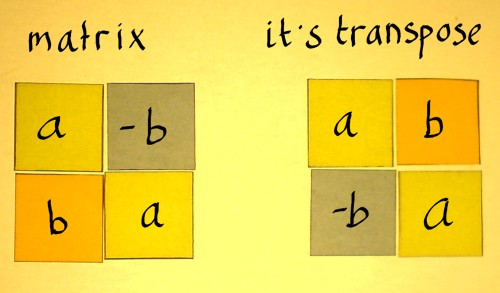# Java Program To Display Transpose Matrix | 3 Ways

Java Program to display/print the transpose of a given matrix.  The following program to print the transpose of a matrix has been written in three different possible ways which have been shared here:

• Using For Loop
• Using While Loop
• Using Do-While Loop

The compiler has been added to the aforementioned, so that you can execute the programs, along with suitable examples and sample outputs.

The Transpose of a given matrix is an operator which flips it over its diagonal. The order of the matrix changes unless it is a square matrix. The transpose of a transpose of a matrix is the given matrix itself – Check to Add two matrices.

Mathematically, [AT]TA## Using For Loop

1) Transpose matrix will formed by inter changing the rows and columns of the original matrix .

2) Read the row number and column number and insert the elements in to the array mat1[][] using two for loops. for i=0 to i<row. for j=0 to j<col. insert element at mat[i][j].

3) To transpose the matrix, row>col then n=row /row<col then n=col. for  i= 0  to  i < n. for  j= i+1  to j < n. Interchange the elements a[i][j] to a[j][i] and vice versa.

4) Print the transpose matrix using two for loops. for  i=0  to i < col. for  j=0 to  j< row. print mat[i][j].

Output:

### Using While Loop

1) Insert the elements into the matrix mat1 using while loop.

a) The while loop iterates until i<row increases the i value. Inner loop iterates until the condition j<col is false. Insert the element at mat1[i][j]. Increase the j value.

2) Now We are finding the transpose of mat1[][]. If row>col then n=row otherwise n=col. while loop iterates until i<n. j=i+1. while loop iterates until j<n. interchange the elements mat1[j][i] to mat1[i][j] vise versa. increase the j value. increase the i value.

3) Print the transpose of a matrix using two while loops with conditions i < col, j < row.

Output:

#### Using Do-While Loop

1) To insert the elements into the matrix,

a) i=0 do loop

• j=0 do loop
• insert an element at mat1[i][j], increase the j value
• then check the condition. This loop iterates until j<col.
• increase the i value. Then check the condition i<row. This loop iterates until i<row.

2) To transpose the matrix-   row>col then n=row else n=col. i=0. do loop j=i+1. do loop. interchange the elements  mat1[i][j] to mat1[j][i], increase the j value. Check the condition j<n at while.

This loop iterates until i<n condition is false. Increase the i value. Then check the condition i<n at while. This loop iterates until condition i<n is false.

3) Print the transpose of the matrix using two do the loop where inner loop checks the condition j<row, outer loop checks the condition i<col.

More Java Programs:

x

## Plus Star Pattern Java Program | Patterns

Java program to print plus star pattern program – We have written the below print/draw ...